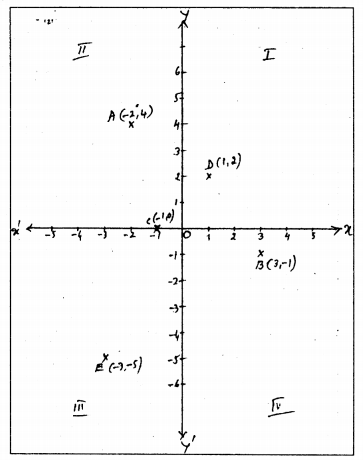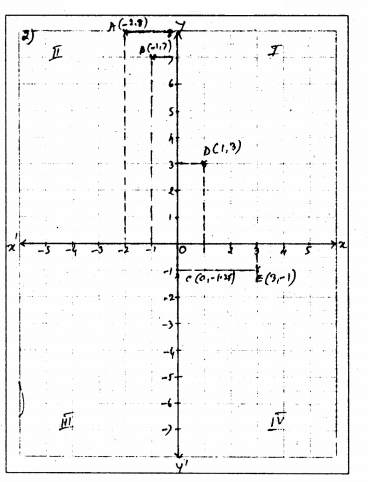# KSEEB Solutions for Class 9 Maths Chapter 9 Coordinate Geometry Ex 9.3

KSEEB Solutions for Class 9 Maths Chapter 9 Coordinate Geometry Ex 9.3 are part of KSEEB Solutions for Class 9 Maths. Here we have given Karnataka Board Class 9 Maths Chapter 9 Coordinate Geometry Exercise 9.3.

## Karnataka Board Class 9 Maths Chapter 9 Coordinate Geometry Ex 9.3

Question 1.
In which quadrant or on which axis do each of the points (-2, 4), (3, -1), (-1. 0), (1, 2) and (-3, -5) lie? Verify your answer by locating them on the Cartesian plane.
Solution:C (-1, 0) Negative x-axis

Question 2.
Plot the points (x, y) given in the following table on the plane, choosing suitable units of distance on the axes.

 x -2 -1 0 1 3 y 8 7 -1.25 3 -1

Solution:
A                            B                              C                                D                                 E

 x -2 -1 0 1 3 y 8 7 -1.25 3 -1A (-2, 8)
B (-1, 7)
C (0,-1.25)
D (1, 3)
E (3, -1)

We hope the KSEEB Solutions for Class 9 Maths Chapter 9 Coordinate Geometry Ex 9.3 helps you. If you have any query regarding Karnataka Board Class 9 Maths Chapter 9 Coordinate Geometry Exercise 9.3, drop a comment below and we will get back to you at the earliest.